MORE IN Engineering Mechanics (EM)
SPPU First Year Engineering (Semester 2)
Engineering Mechanics (EM)
December 2014
Total marks: --
Total time: --
INSTRUCTIONS
(1) Assume appropriate data and state your reasons
(2) Marks are given to the right of every question
(3) Draw neat diagrams wherever necessary

Answer any one question from Q1 and Q2
1 (a) Determine the magnitude of force P so that the resultant of the force system as shown in Fig. 1a is vertical and hence find magnitude of resultant force.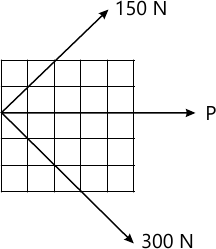4 M
1 (b) The small collar of mass 0.5 kg is released from rest at A and strikes the base B with velocity 4.7 m/s as shown in Fig. 1 b, determine the work done by frictional force using work energy principle.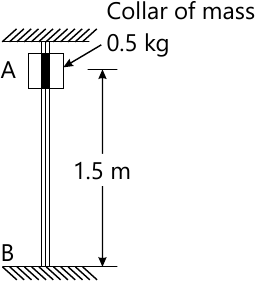4 M
1 (c) During a break test, the car of mass 1500 kg is stop from an initial speed of 100 kmph in a distance of 50 m. Determine the breaking force assuming uniform deceleration.
4 M
1 (d) A particle moves in a circular path of radius 0.4m. Calculate magnitude of acceleration a of the particle if its speed is 0.6 m/s but it increasing at the rate of 1.2 m/s each second.
4 M

2 (a) The pendulum bob has a mass m and is released from rest when θ= 0°. Determine the tension in the cord as a function of the angle of decent θ. Neglect the size of bob.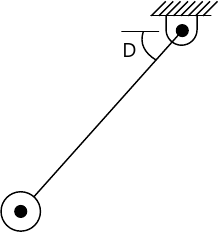4 M
2 (b) Determine the coordinate of centroid of the shaded area as shown in Fig, 2b.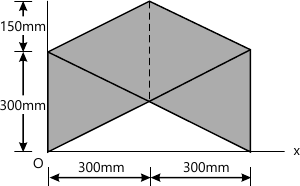4 M
2 (c) A ball is projected vertically upward with a velocity of 9.81 m/s. Determine the maximum height travel by the ball, the velocity at which it strikes the ground and total time of journey.
4 M
2 (d) One of the requirement for tennis balls to be used in official competition is that, when dropped onto a rigid surface from a height of 2540 mm, the height of the first bounce of the ball must be in the range of 1346 mm ≤ h ≥ 1473 mm. Determine the range of the coefficient of restitution of the tennis balls satisfying this requirement.
4 M

Answer any one question from Q3 and Q4
3 (a) A cylinder of weight 1000 N is rest on the stair as shown in Fig, 3 a. Determine the minimum magnitude of force P to raise the cylinder over the steps.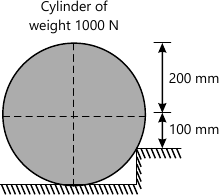6 M
3 (b) The ball is suspended from the horizontal ring using three spring each having a stiffness of k = 50 N/m and an unstretched length of 1.5 m. If h = 2 m, determine the weight of ball. Refer Fig. 3 b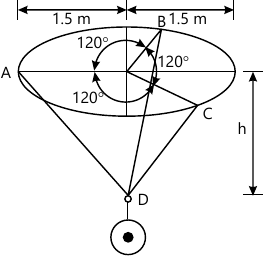6 M
3 (c) Determine the length a of overhang so that the reaction at B is twice of the reaction at A for the beam loaded and supported as shown in Fig. 3 c.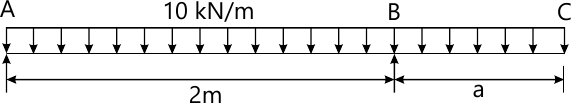5 M

4 (a) Three parallel bolting forces act on the rim of the circular cover plate as shown in Fig. 4 a. Determine the magnitude, direction and locate point of application of the resultant force on the cover plate.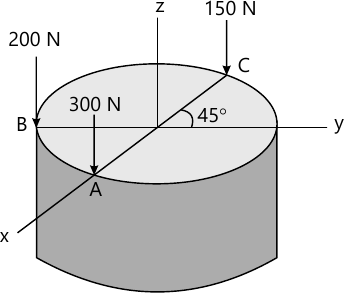6 M
4 (b) Determine the magnitude and direction θ of force F so that the particle is in equilibrium. Refer Fig. 4 b.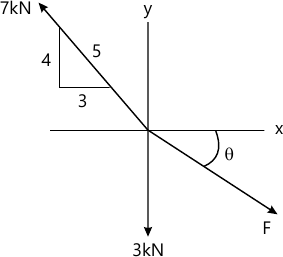5 M
4 (c) A force of 150 N acts on the end of beam ABD as shown in Fig. 4 c. Determine the magnitude of tension in cable BC to maintain equilibrium.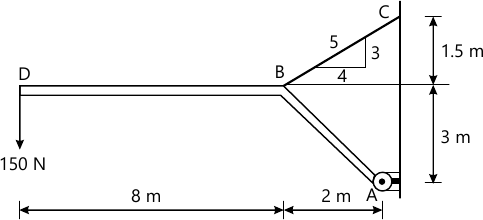6 M

Answer any one question from Q5 and Q6
5 (a) A block of mass m rest on a frictional plane which makes an angle α with the horizontal as shown in Fig. 5 a. If the coefficient of friction between the block and the frictional plane is 0.2, determine the angle α for limiting condition.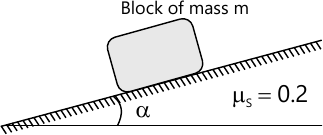5 M
5 (b) Determine the forces in each member of the plane truss as shown in Fig. 5 b in teRMS of the external loading and state if the members are in tension or compression. Use θ = 30°, L = 2 m and P = 100 N.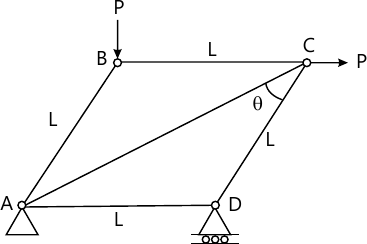6 M
5 (c) Determine the range of P for the equilibrium of block of weight W as shown in Fig. 5 c. The coefficient of friction between rope and pulley is 0.2.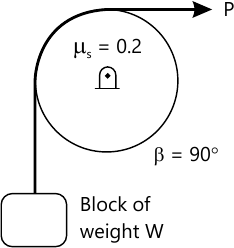6 M

6 (a) Determine the components of reaction at C for the frame loaded and supported as shown in Fig. 6 a.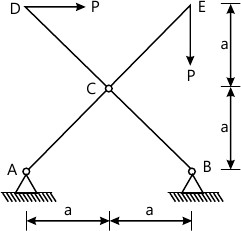6 M
6 (b) Determine the range of P for the limiting equilibrium of block B of mass 150 kg rest on an inclined plane as shown in Fig. 6 b.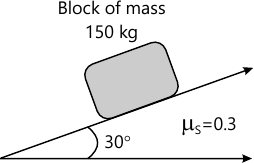6 M
6 (c) State the assumption for the analysis of cable and laws of static friction.
5 M

More question papers from Engineering Mechanics (EM)